Next: Complexity Up: Algorithms for the Previous: Contents

# Introduction

An ordered set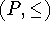is a pair of a set P and a reflexive, antisymmetric and transitive relation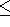on P. We will usually omit the pair notation and call P an ordered set also. We will call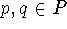comparable   and write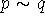iff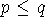or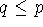. Every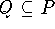is an ordered set with the induced order from P and will be called an ordered subset of P.   All subsets of ordered sets will be viewed as ordered subsets. A subset S of an ordered set P has an upper bound x (denoted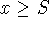)   iff for all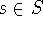we have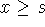.   y is the supremum   or lowest upper bound of S (denoted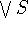)   iff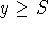and for allwe have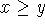. Lower bounds  and infima  resp. greatest lower bounds (denoted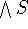)  are defined dually. An ordered set is a chain iff any two points in it are comparable.   A mapping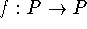is called order-preserving   iff for allwe have thatimplies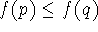. An ordered set P is said to have the fixed point property   iff each order-preserving self maphas a fixed point, i.e., there is a point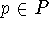with   p=f(p).   An order-preserving map without a fixed point is called fixed point free.
The main object of this paper is the following - somewhat vague - open question that is for example to be found on ORDER's problem list.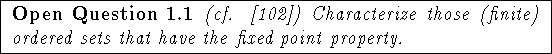The recorded history of this problem seems to start in the papers  by Knaster in the twenties and then in  by Tarski and  by Davis in the fifties, where the question is answered for lattices (a lattice   is an ordered set in which any finite subset has a supremum and an infimum, it is complete   iff every subset has a supremum and an infimum): A lattice has the fixed point property iff it is complete (also cf. , Exercise 1J, Tarski's remarks in  and the remarks in  footnote 2 on p. 284). After that there were papers by Abian and Brown ()   and Pelczar (), which recorded one of today's standard tools: If P is a chain-complete ordered set (i.e., every nonempty chain has a supremum and an infimum),is order-preserving and there is asuch that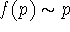, then f has a fixed point. Problem 1.1 seems to have been first raised in the text  by Crawley and Dilworth. The next milestone then is Rival's characterization of the fixed point property for finite ordered sets of height 1 in . Since then the fixed point problem has drawn more and more attention in many papers as the list of references shows. The vague formulation of the problem has inspired many possible approaches. Among them are the following
1. One can try as in the above mentioned theorems to characterize the fixed point property for certain classes of ordered sets. This is done for example by Fofanova and Rutkowski in  (sets of width 2), by Höft and Höft in - (lexicographic sums), by Rival as mentioned in  (sets of height 1), by Ewacha and Rival in , Rutkowski in , the author in  (``small" sets), Davis and Tarski in  and  (lattices) and possibly most importantly by Roddy in , where the fixed point problem is solved for finite products of finite ordered sets with a beautiful argument.
2.   One can prove fixed point theorems that do not necessarily handle an established class of ordered sets, but that provide new insights through possible reductions (for example done by Constantin and Fournier in , by Rival in , and by the author in , ) or through the identification of substructures that force the fixed point property (done for example by Baclawski and Björner in , by Edelman in , and by Rutkowski in ).
3.   One can try to prove results about forbidden retracts, which can be done in special cases (for example by Fofanova and Rutkowski in , or by Rutkowski and the author in ), but which might be too complicated in general (cf. section 4.8).
4.   One can relate the problem to its analogue in topology. (When does a topological space have the fixed point property?) This is done for example by Baclawski and Björner in , and by Constantin and Fournier in .
5.   One can devise algorithms that check whether an arbitrary finite ordered set has the fixed point property (done for example by Pickering, Roddy and Stadel in  and by Xia in ).
Approach 5 leads to the question how efficient such an algorithm might be, on which we focus in section 2. We will demonstrate that the efficiency problem is very similar to other decision problems that are NP-complete. Shortly before the deadline for this article the author received the preprint  by Duffus and Goddard in which it is shown that the decision problem 2.3 is NP-complete (cf. Definition 2.12).  is still being refereed as this is written. The author considers the arguments given in  valid and can thus no longer consider problem 2.3 open in its full generality. Efficient algorithmic approaches to the problem are still interesting, as they now actually have a wider scope than before. Also there are still interesting classes of ordered sets for which the complexity of problem 2.3 is not known (e.g., truncated lattices, ordered sets of height 2 or width 3). We will discuss the algorithmic ramifications of approach 2 of reducing the ordered set in section 3 and of approach 4 of relating the problem to topology in section 4. We devote section 5 to the interesting ``spin-offs" that the investigation of the fixed point property has produced. Proofs that were omitted were either considered simple by the author or a reference to the proof is given. Sections 2, 3 and 4 are (aside from references to related results) independent of each other and can be read in any order.Next: Complexity Up: Algorithms for the Previous: Contents

Bernd.S.W.Schroder